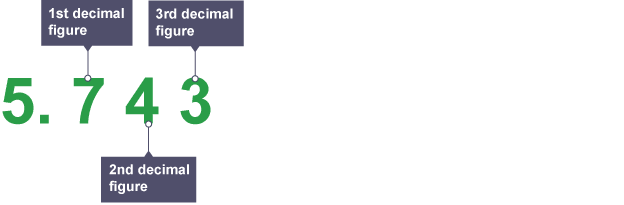Maths Support Week 4 Summer 1

Next week the children will be focussing on rounding within their Maths. The children will round decimals with two decimal places to the nearest whole number and to one decimal place. They will also read, write, order and compare numbers with up to three decimal places.

Rounding to a given number of places

Counting decimal places

Decimal places are counted from the decimal point:So, the numberhas four decimal places, whilehas two decimal places.

To round a number to a given number of decimal places, look at the number in the next decimal place:

• If it’s less than, round down
• If it’sor more, round up

Example

Roundtodecimal places

This means we needdigits after the decimal point.Because the next digit, is more than, we round theup.(decimal places).

What We’ve Learnt This Week!

This week we have learnt all about fractions with different denominators and how to compare and order them, which was lots of fun! We have also been working towards writing a newspaper report about the event in the Porsche showroom from the book ‘Cosmic’. In PE, we learnt how to bowl correctly in a game of rounders in order for it to be a good ball, which is above the knees and below the head.

I’m really looking forward to next week!

From Freya.

Class Attendance=99%

Maths Support Summer 1 Week 3

Next week, the children will focus on solving problems involving converting between units of time.

Time problems

Follow these tips when solving time problems:

• If some of the times in the problem are in seconds, some in minutes and some in hours, change some of them so they are either all in seconds, all in minutes or all in hours.
• When reading timetables, make sure you know what type of information is in each column and row.

Example 1

How long does it take for the 11.55 train from Normington to get to Kirkfield?(The empty space in the table means the 10:39 train from Normington doesn’t stop at Baskwell.)

Look along the Normington row until you reach the column that starts with the 11.55.

Look down that column until you get to Kirkfield and read off the time, 12.44.

Now you need to find the difference between 11.55 and 12.44. A good way to do this is to break up the time into smaller units within each hour.5 + 44 = 49 minutes.

So the 11.55 train from Normington takes 49 minutes to get to Kirkfield.

Example 2

Sammy wakes up at 7.00am. He eats his breakfast at 7.30am and sets off for school at 8.30am. School starts at 9.00am.

First break is at 10.30am and by 12.00pm in the afternoon he is ready for lunch. School finishes at 3.30pm when Sammy is picked up by his Dad. He eats tea at 5.00pm and then leaves for football practice, which starts at 6.00pm.

When he gets home at 7.30pm, he has a bath and a snack, making his way up to bed at 8.30pm so he is bright and fresh for school in the morning.

Maths Support Summer 1 Week 2

Next week, the children will be covering fractions. They will be comparing and ordering fractions whose denominators are all multiples of the same number.

Adding and subtracting fractions

If you were to addand, it is hard to picture what the answer would be. Rewriting the fractions with a common bottom number, or denominator (in this case,), makes it easier.

Remember, you can only add and subtract fractions when the bottom numbers, or denominators, are the same.

So, to add or subtract fractions:

1. Change the fractions so they have the same denominator.
2. Add or subtract the top numbers, or numerators.

Example